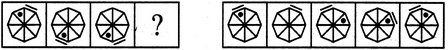Non Verbal Reasoning - Analogy - Discussion

Discussion :: Analogy - Section 1 (Q.No.17)

Each of the following questions consists of two sets of figures. Figures A, B, C and D constitute the Problem Set while figures 1, 2, 3, 4 and 5 constitute the Answer Set. There is a definite relationship between figures A and B. Establish a similar relationship between figures C and D by selecting a suitable figure from the Answer Set that would replace the question mark (?) in fig. (D).

17.

Select a suitable figure from the Answer Figures that would replace the question mark (?).

Problem Figures:                            Answer Figures:(A)     (B)      (C)     (D)                  (1)      (2)      (3)      (4)      (5)

 [A]. 1 [B]. 2 [C]. 3 [D]. 4 [E]. 5

Explanation:

The line segment moves four spaces ACW and the dot moves three spaces ACW.

 Sachin said: (Feb 23, 2015) How it could be from B to C? here the segment does not move four spaces ACW or the dot had not moved, even a spaces.

 Keerthi Kumar said: (Jul 11, 2015) There is a definite relationship between figures A and B. Establish a similar relationship between figures C and D by selecting a suitable figure from the Answer Set that would replace the question mark (?) in fig. (D).

 Jitu said: (Oct 5, 2016) If we consider both line and dot is moving CW then as per A&B's relationship its moving 4 steps CW. And hence E is also a correct answer.

 Siva Raja said: (Mar 12, 2017) I Can't understand. Please explain.

 Kavya said: (Jul 27, 2017) Dot moves 3 places backwards similarly the line moves four steps back.

 Harsha said: (Nov 17, 2017) Observe figure A & B separately and then find figure D for C having similar relation.

 Pallavi said: (Feb 1, 2018) The answer will be D.

 Sharanu B Koti said: (Feb 20, 2018) What is ACW?

 Pankaj Verma said: (Mar 5, 2018) The correct answer is D.

 Judy B said: (May 8, 2018) Number 1 is also the correct answer. The line goes to opposite and the dot flips on the horizontal. 1 matches that pattern.